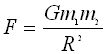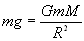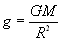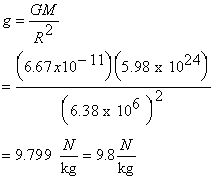#### Calculating g using the Law of Universal GravitationRecall that g around the surface of the Earth is a constant with a calculated value of  9.8 m/s2 or  9.8 N/Kg

Another way to calculate the acceleration due to gravity g is by using Newton's Law of Universal Gravitation

Recall that the Force of gravity around the surface of the Earth is  calculated with the formula F= m x g

But we can also use Newton's Law of Universal Gravitation to calculate the value of g.Recall that In the above equation F is the force of gravity,  You can also call it FgAlso recall that Fg = m g

Thus the two expressions can be equated to one another' and we can say thatwhere:

• m is the mass of any object at the surface of the Earth.
• M is the mass of the Earth
• G is the constant of Universal Gravitation (6.67 x 10-11 Nm2/kg2).Note that the mass m can be dropped out from each side of the equation and we can simplify the above as:The value of g on Earth can be calculated if we know the mass of the Earth and its radius R.This fact should not surprise us after all we've always stated that the acceleration due to gravity on an object is independent of the object's mass.

##### Example:

Use the information that the mass of the Earth is 5.98 x 1024 kg and that its radius is 6.38 x 103 km  (6.38 x 10 m) to confirm that g on Earth is approximately 9.8 N/kg

Solution:

Using the formula above we have:Do a unit analysis check on your own to verify that the answer is in N/kgIt's always a good practice to do this at the end of every problem to verify that the units are correct!